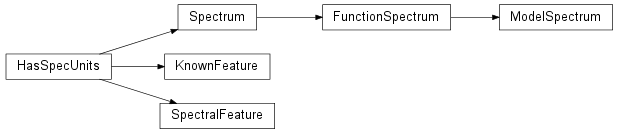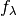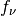# spec – spectrum and SED classes and tools¶

The spec module contains classes and funtions focused on plotting and analysis of arbitrary spectra and SEDs, as well as related utility functions.

Defaults tend to be oriented towards optical, but everything should still be valid in other bands.

The obstools module
Includes tools for spectra such as extinction/redenning corrections.
The phot module
Allows photometric bands to be cross-calibrated to spectra (and vice versa)
The io module

## Classes and Inheritance Structure¶## Module API¶

class astropysics.spec.FunctionSpectrum(xi, fluxf, errf=None, ivarf=None, unit='wl')

This is a Spectrum generated by functional forms rather than using fixed samples. Setting x will determine where the function is evaluated (and do the evaluation).

err
flux
ivar
x
class astropysics.spec.HasSpecUnits(unit)

Bases: object

This class is a mixin superclass for objects that have spectral-like units (e.g. flux spectral densityor, luminosity spectral density, etc.). It adds a property unit that can be set with a string to change the units of the object.

The following steps be done by subclasses:

1. override method HasSpecUnits._applyUnits() - see method doc for details.
2. call HasSpecUnits.__init__() with the unit argument providing the units of the initial input data.
static strToUnit(typestr)

converts a unit string into a standardized form

returns phystype,unit,scaling (scaling relative to cgs)

unit
class astropysics.spec.KnownFeature(loc, name='', strength=None, unit='wavelength')

Represents a spectral feature with known location to be matched to an unknown feature (or included in line lists).

The strength attribute can be positive to imply emmission, negative to mean absorption, or None to leave unspecified

getUnitLoc(unit)

gets the location in the specified units (without changing the current unit)

class astropysics.spec.ModelSpectrum(xi, model, noisetype=None, noisefunc=None, unit='wl')

A Spectrum that follows the provided astropysics.models.FunctionModel1D

noisefunc should be of the form nf(x,flux)

noisetype determines the noise model - can be:

• None

no noise

• ‘poisson’

poisson noise where the noisefunc determines the poissonian scale factor

• ‘normal’ or ‘gauss’

normally-distributed noise where the noisefunc determines sigma

fitToSpectrum(spec, **kwargs)

Fit the model parameters to match this Spectrum to the supplied Spectrum

kwargs are passed into the fitData method of the model

model
noisefunc
noisetype
plotModels(*args, **kwargs)

calls the model’s plot method with args and kwargs

x
class astropysics.spec.SpectralFeature(extent, unit='wavelength', continuum='fromspec')

This class represents a Spectral Feature/line in a Spectrum.

All SpecralFeatures have a rest value,observed value (possibly with error), flux value (possibly with error), and equivalent width (possibly with error)

Note that equivalent width is always expected to be in angstroms

center

central wavelength/frrequency/energy of this feature

computeFeatureData(spec, fit=False, interactive=False, edges='interp')

computes the data (center,flux, equivalent width, etc.) for this feature

if fit is true, it specifies a model that should be used to fit the feature.

if interactive is True, a fitgui will be displayed to fit the data. if fit is False, interactive will be ignored

edges determine the behavior between the last pixel and the extent edge:

• ‘tweak’: adjust the extent to the nearest pixel and recompute
• ‘interp’: interpolate the flux where the edges
• None/False/’‘: Do nothing
identify(nameorknown)
idname

name string of the identity of this feature

class astropysics.spec.Spectrum(x, flux, err=None, ivar=None, unit='wl', name='', copy=True, sort=True)

Represents a flux/luminosity/intensity

Note that operations are performed in-place, and properties retrieve the same versions that are changed (except ivar)

sets the x-axis values and the flux. Optionally, an error can be given by supplying an error or an inverse variance (bot not both)

copy determines if the inputs will be copied if they are already arrays

sort determines if the array values will be sorted by x

add a spectral feature at the provided x-location. Edges are inferred from either the continuum zero-point (if continuum is present) or where the derivative changes sign

If window is specified, it is the number of pixels to check for the edge.

If smooth is not None, it specifies a smoothing size to apply - positive for gaussian sigma or negative for boxcar half-width.

Adds a spectral feature to the feature list for this spectrum.

Parameters: lower (scalar) – The lower spectral x-axis bound for the feature. upper (scalar) – The upper spectral x-axis bound for the feature. continuum – See Spectrum.computeFeatureData() for details. identity – If not None, the feature will be identified - see SpectralFeature.identify() for valid values. The SpectralFeature object
computeFlux(bands, **kwargs)

this computes the flux of the spectrum in the provided bands

bands can be a sequence of band objects or strings which will be mapped to bands through the phot.bands registry

kwargs are passed into phot.Band.computeFlux

computeMag(bands, **kwargs)

this computes the magnitude of the spectrum in the provided bands

bands can be a sequence of band objects or strings which will be mapped to bands through the phot.bands registry

kwargs are passed into phot.Band.computeMag

copy()

Generates a deep copy of this Spectrum

err
features

The spectral features in this spectrum

fitContinuum(model=None, weighted=False, evaluate=False, interactive=False, **kwargs)

this method computes a continuum fit to the spectrum using a model from astropysics.models (list_models will give all options) or an callable with a fitData(x,y) function

if model is None, the existing model will be used if present, or if there is None, the default is ‘uniformknotspline’. Otherwise, it may be any acceptable model (see models.get_model_class())

kwargs are passed into the constructor for the model

if weighted, the inverse variance will be used as weights to the continuum fit

if interactive is True, the fitgui interface will be displayed to tune the continuum fit

the fitted model is assigned to self.continuum or evaluated at the spectrum points if evaluate is True and the results are set to self.continuum

flux
getDlogx(mean=True, logbase=10)

get the logarithmic spacing of the x-axis, which is always 1 element shorter than x

if mean, returns the mean of the spacing

getDx(mean=True)

get the spacing of the x-axis, which is always 1 element shorter than x

if mean, returns the mean of the spacing

getPhotonFlux()

returns flux adjusted to represent photons s^-1 cm^-2 xunit^-1

getUnitFlux(units, err=False)

returns x and flux of this spectrum in a new unit system without changing the selected unit

err can be False, True or ‘ivar’

if err is False, returns x,flux if err is True, returns x,flux,err if err is ‘ivar’, returns x,flux,ivar

getZQualStr()
isLinear(eps=1e-10)

Determines if the x-spacing is linear (e.g. evenly spaced so that dx ~ constant with a standard deviation of eps)

isLogarithmic(eps=1e-10)

Determines if the x-spacing is logarithmic (e.g. evenly spaced in logarithmic bins so that dx ~ x to tolerance of eps)

isXMatched(other, tol=1e-10)

tests if the x-axis of this Spectrum matches that of another Spectrum or equal length array, with an average deviation less than tol

ivar
k = -1
linearize(lower=None, upper=None, **kwargs)

convinience function for resampling to an equally-spaced linear x-axis

if lower or upper are None, the upper and lower x values are used kwargs go into Spectrum.resample

Load a saved Spectrum from the given file

logify(lower=None, upper=None, **kwargs)

convinience function for resampling to an x-axis that is evenly spaced in logarithmic bins. Note that lower and upper are the x-axis values themselves, NOT log(xvalue)

if lower or upper are None, the upper and lower x values are used

nflux
normalizeByContinuum()

Divide by the flux by the continuum

npix
plot(fmt=None, ploterrs=0.1, plotcontinuum=True, smoothing=None, step=True, clf=True, colors=('b', 'g', 'r', 'k'), restframe=True, xrng=None, **kwargs)

Use matplotlib to plot the Spectrum object. The resulting plot shows the flux, error (if present), and continuum (if present).

If step is True, the plot will be a step plot instead of a line plot.

smoothing is passed into the Spectrum.smooth() method - see that method for details.

colors should be a 3-tuple that applies to (spectrum,error,invaliderror,continuum) and kwargs go into spectrum and error plots.

If restframe is True, the x-axis is offset to the rest frame.

If ploterrs or plotcontinuum is a number, the plot will be scaled so that the mean value matches the mean of the spectrum times the numeric value. If either are True, the scaling will match the actual value. If False, the plots will not be shown.

xrng can specify the range of x-values to plot (lowerx,upperx), or can be None to plot the whole spectrum.

kwargs are passed into either the matplotlib.pyplot.plot() or matplotlib.pyplot.step() function.

plotSpylot(spylotinstance=None, show=True)

Displays the spectrum using the astropysics.gui.spylot spectrum plotter.

Parameters: spylotinstance – If None, a new instance of Spylot will be generated with this Spectrum as the only Spectrum. If it is a Spylot instance, this Spectrum will be added to the end of the list on that instance. show – If True and spylotinstance is None, the GUI will be shown using enthought.traits.has_traits.HasTraits.edit_traits() (kwargs will be passed to edit_traits() if show is a dictionary), If a spylotinstance is provided, the GUI for that instace will be switched to this Spectrum. Otherwise, nothing will be done to the GUI. A Spylot instance.
rejectOutliersFromContinuum(sig=3, iters=1, center='median', savecont=False)

rejects outliers and returns the resulting continuum. see utils.sigma_clip for arguments

if savecont is True, the outlier-rejected value will be saved as the new continuum

removeFeatureLocation(loc)

removes the feature with a center nearest to the requested location

raises IndexError if there are no features

removeSpectralFeature(iorname)

remove the requested spectral feature, either by index or by line name

resample(newx, interpolation='linear', replace=True, **kwargs)

this interpolates the flux to populate the supplied x-axis

kwargs go into the interpolation routine as described below

interpolations can be: ‘linear’: simple linear interpolation ‘spline’: a k-order spline with smoothing factor s is used, where s and k are set by kwargs. if the ‘save’ kwarg is True, the spline is saved and will be used for subsequent resamplings. if ‘clear’ is True, the existing spline will be cleared and a new spline will be calculated. note that default spline has smoothing=0, which interpolates through every point

WARNING: this does not treat the errors properly yet - currently just interpolates

revertContinuum()

Revert to flux before continuum subtraction

rgbEyeColor()

This uses the ‘eye’ group in phot.bands to convert a spectrum to an (r,g,b) tuple such that (1,1,1) corresponds to a T=5800 blackbody spectrum (approximating the sun).

save(fn, **kwargs)

Save this Spectrum as a pickled object with the provided file name. kwargs are passed into utils.fpickle

shape
smooth(width=1, filtertype='gaussian', replace=True)

smooths the flux in this object by a filter of the given filtertype (can be either ‘gaussian’ or ‘boxcar’/’uniform’). Note that filtertype can also be None, in which case a gaussian filter will be used if width>0, or boxcar if width<0.

if replace is True, the flux in this object is replaced by the smoothed flux and the error is smoothed in the same fashion

width is in pixels, either as sigma for gaussian or half-width for boxcar

returns smoothedflux,smoothederr

subtractContinuum()

Subtract the continuum from the flux

v = 'unknown'
x

x-axis as measured

x0

x-axis in rest frame

zqual
astropysics.spec.air_to_vacuum(airwl, nouvconv=True)

Returns vacuum wavelength of the provided air wavelength array or scalar. Good to ~ .0005 angstroms.

If nouvconv is True, does nothing for air wavelength < 2000 angstroms.

Input must be in angstroms.

Adapted from idlutils airtovac.pro, based on the IAU standard for conversion in Morton (1991 Ap.J. Suppl. 77, 119)

astropysics.spec.align_spectra(specs, ressample='super', interpolation='linear', copy=False)

resample the spectra in the sequence specs so that they all have the same x-axis.

ressample can be ‘super’ lower-resolution spectra to the highest or ‘sub’ to interolate the highest-resolution spectrum to the lowest alternateively, ‘logsuper’ or ‘logsub’ will use the logarithmic resolution to determine

copy makes new copies of the Spectrum objects

returns specs, or new copies if copy is True

astropysics.spec.build_model_spectrum(n=1024, T=5800, range=None, peak=1, z=0, noise=None, lines=None, linesampling=1, name='Model')

Constructs a basic spectral object composed of a blackbody with temperature T, a set of lines, and noise.

peak sets the peak of the blackbody curve, z is the redshift.

noise can be:

• positive

additive gaussian noise with the specified amplitude

• negative

multiplicative uniform noise with the specified amplitude

• True

poisson

• False/None

no noise

lines can be None or a sequence, where each element of the sequence is:

• a 3-sequence (location,amplitude,width) where if width is positive it gives a gaussian sigma, or negative gives a lorentzian FWHM
• a 4-sequence (location,amplitude,sigma,FWHM) to use a voigt profile
• a FunctionModel1D for the line flux

linesampling is passed into FunctionModel1D.pixelize or the models will be called directly if it is 1

this converts an integer pixel lag for a given x-axis into a redshift

currently the only supported unit for the x-axis is angstroms

avgbad replaces undeterminable velocities with the average of the others, else they are set to 0

Generates a spectral line list.

lst can be:

• either ‘galaxy’ or ‘stellar’ to load built-in line lists of common optical features
• a filename for a file with one spectral feature per line - either one column of the spectral location, two columns (location,name), or three columns (location,name,strength)
• a sequence of KnownFeature objects.

tol is the seperation between locations allowed until two features are considered duplicates (or None to require an exact match)

ondup specifies the action to take if a duplicate is encountered:

• ‘raise’:raise an IndexError
• ‘warn’: issue a warning
• ‘remove’:removes all but last in a set of duplicates
• None: ignore

The list will be sorted in increasing (decreasing) order if sort is positive (negative), or no sorting will be done if it is 0/False

returns a list of KnownFeature objects

astropysics.spec.vacuum_to_air(vacwl, nouvconv=True)

Returns air wavelength of the provided vacuum wavelength array or scalar. Good to ~ .0005 angstroms.

If nouvconv is True, does nothing for air wavelength < 2000 angstroms.

Input must be in angstroms.

astropysics.spec.zfind(specobj, templates, lags=(0, 200), checkspec=True, checktemplates=True, verbose=True, interpolation=None)

computes the best fit by linear least-squares fitting of templates to the spectrum for each possible pixel offset. Weighted fits will be done if the spectrum ivars are different.

lags can either be a sequence of lags or a 2-tuple specifying the lower and upper possible lags

specobj must be a Spectrum object or a sequence of (flux,[x],[ivar]) or flux. If it is not logarithmically spaced, it will be interpolated

templates can be either a sequence of Spectrum objects or an array with at least one dimension matching the pixel dimension. (Note that templates longer than the spectrum will not use information off the edges)

interpolation is the technique for interpolating for sub-pixel lags. If None, no interpolation is used, so lags must be integers (but this method is much faster)

returns besti,lags,zs,coeffs,xs,fitfluxes,rchi2s

HasSpecUnits._applyUnits(xtrans, xitrans, xftrans, xfinplace)

This method must be overridden by subclasses. The overriding method should use the xftrans OR xfinplace (not both) to convert the old x-axis and flux axis into new x axes and flux values

xtrans and xitrans are of the form newx = xtrans(oldx) and oldx = xitrans(newx)

xftrans is a function with the signature newx,newf = xftrans(oldx,oldf) x is expected to be 1D, and f is expected to be either 1D or must have last dimension equal to len(x)

xfinplace(x,f) returns None but uses in-place operators to adjust the values of x and f